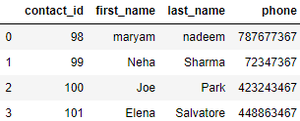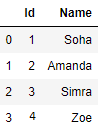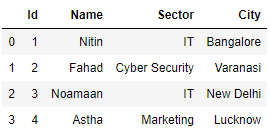# Read SQL database table into a Pandas DataFrame using SQLAlchemy

• Last Updated : 17 Aug, 2020

To read sql table into a DataFrame using only the table name, without executing any query we use `read_sql_table()` method in Pandas. This function does not support DBAPI connections.

Syntax : pandas.read_sql_table(table_name, con, schema=None, index_col=None, coerce_float=True, parse_dates=None, columns=None, chunksize=None)

Parameters :

table_name : (str) Name of SQL table in database.

con : SQLAlchemy connectable or str.

schema :  (str) Name of SQL schema in database to query (if database flavor supports this). Default is None

index_col : List of string or string. Column(s) to set as index(MultiIndex). Default is None.

coerce_float : (bool) Attempts to convert values of non-string, non-numeric objects (like decimal.Decimal) to floating point.  Default is True

parse_dates : (list or dict)

• List of column names to parse as dates.
• Dict of {column_name: format string} where format string is strftime compatible in case of parsing string times or is one of (D, s, ns, ms, us) in case of parsing integer timestamps.
• Dict of {column_name: arg dict}, where the arg dict corresponds to the keyword arguments of pandas.to_datetime() Especially useful with databases without native Datetime support, such as SQLite.

columns : List of column names to select from SQL table. Default is None

chunksize : (int) If specified, returns an iterator where chunksize is the number of rows to include in each chunk. Default is None.

Return type : DataFrame

Example 1 :

 `# import the modules``import` `pandas as pd ``from` `sqlalchemy ``import` `create_engine`` ` `# SQLAlchemy connectable``cnx ``=` `create_engine(``'sqlite:///contacts.db'``).connect()`` ` `# table named 'contacts' will be returned as a dataframe.``df ``=` `pd.read_sql_table(``'contacts'``, cnx)``print``(df)`

Output :Example 2 :

 `# import the modules``import` `pandas as pd ``from` `sqlalchemy ``import` `create_engine`` ` `# SQLAlchemy connectable``cnx ``=` `create_engine(``'sqlite:///students.db'``).connect()`` ` `# table named 'students' will be returned as a dataframe.``df ``=` `pd.read_sql_table(``'students'``, cnx)``print``(df)`

Output :Example 3 :

 `# import the modules``import` `pandas as pd ``from` `sqlalchemy ``import` `create_engine`` ` `# SQLAlchemy connectable``cnx ``=` `create_engine(``'sqlite:///students.db'``).connect()`` ` `# table named 'employee' will be returned as a dataframe.``df ``=` `pd.read_sql_table(``'employee'``, cnx)``print``(df)`

Output :My Personal Notes arrow_drop_up